Скачать презентацию Foundations of Network and Computer Security John Black

• Количество слайдов: 19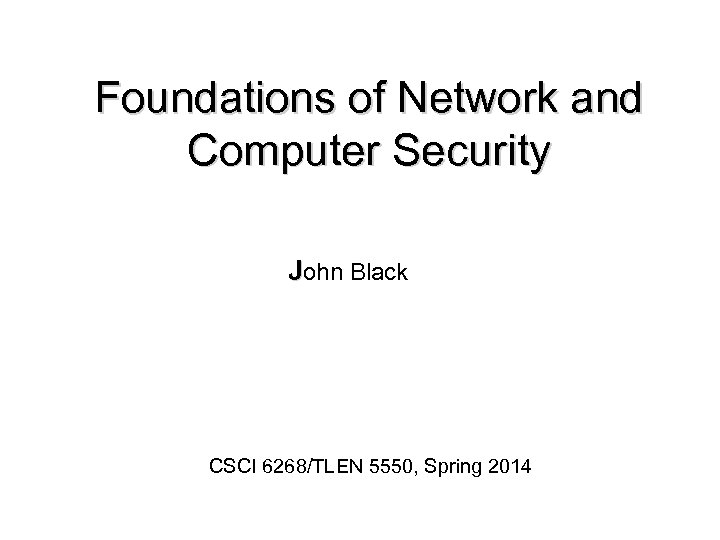Foundations of Network and Computer Security John Black CSCI 6268/TLEN 5550, Spring 2014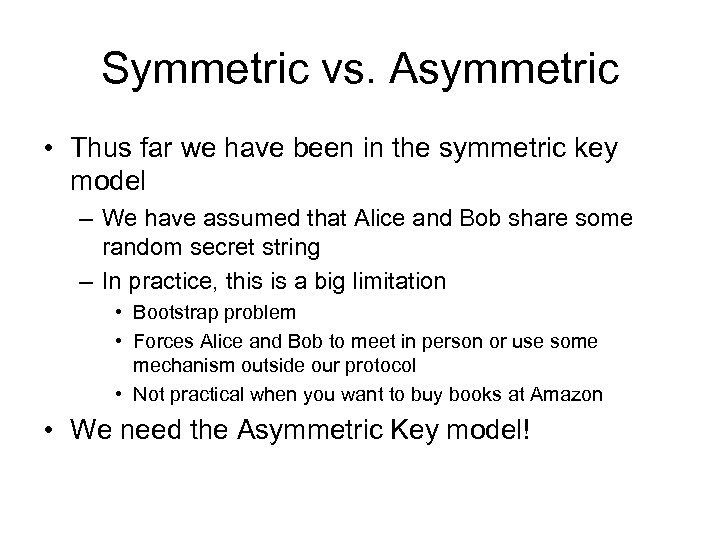Symmetric vs. Asymmetric • Thus far we have been in the symmetric key model – We have assumed that Alice and Bob share some random secret string – In practice, this is a big limitation • Bootstrap problem • Forces Alice and Bob to meet in person or use some mechanism outside our protocol • Not practical when you want to buy books at Amazon • We need the Asymmetric Key model!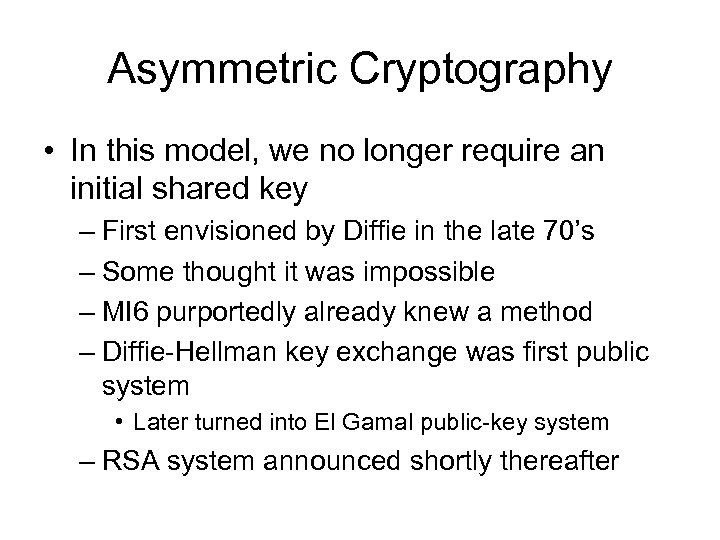Asymmetric Cryptography • In this model, we no longer require an initial shared key – First envisioned by Diffie in the late 70’s – Some thought it was impossible – MI 6 purportedly already knew a method – Diffie-Hellman key exchange was first public system • Later turned into El Gamal public-key system – RSA system announced shortly thereafter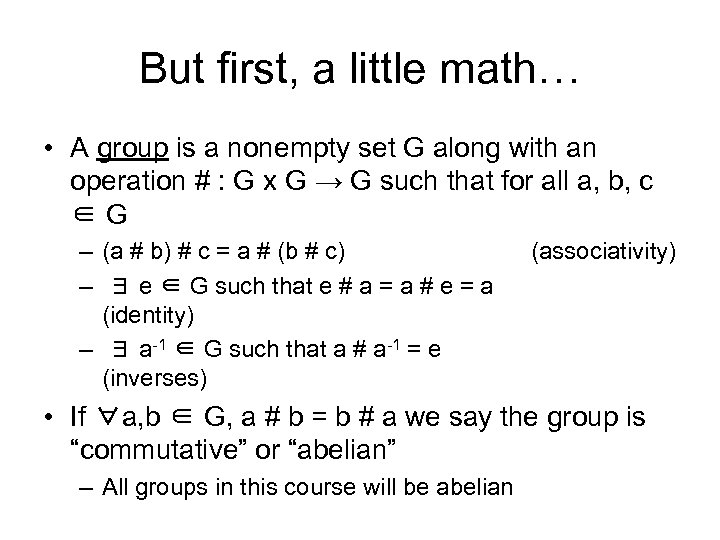But first, a little math… • A group is a nonempty set G along with an operation # : G x G → G such that for all a, b, c ∈G – (a # b) # c = a # (b # c) – ∃ e ∈ G such that e # a = a # e = a (identity) – ∃ a-1 ∈ G such that a # a-1 = e (inverses) (associativity) • If ∀a, b ∈ G, a # b = b # a we say the group is “commutative” or “abelian” – All groups in this course will be abelian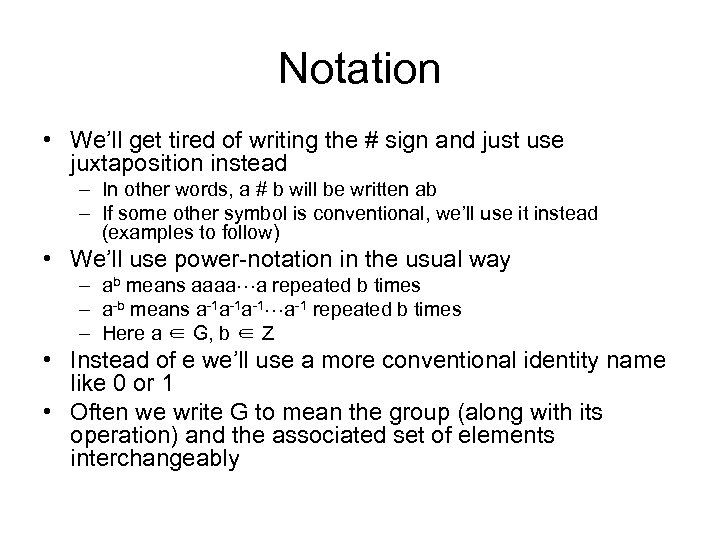Notation • We’ll get tired of writing the # sign and just use juxtaposition instead – In other words, a # b will be written ab – If some other symbol is conventional, we’ll use it instead (examples to follow) • We’ll use power-notation in the usual way – ab means aaaa a repeated b times – a-b means a-1 a-1 a-1 repeated b times – Here a ∈ G, b ∈ Z • Instead of e we’ll use a more conventional identity name like 0 or 1 • Often we write G to mean the group (along with its operation) and the associated set of elements interchangeably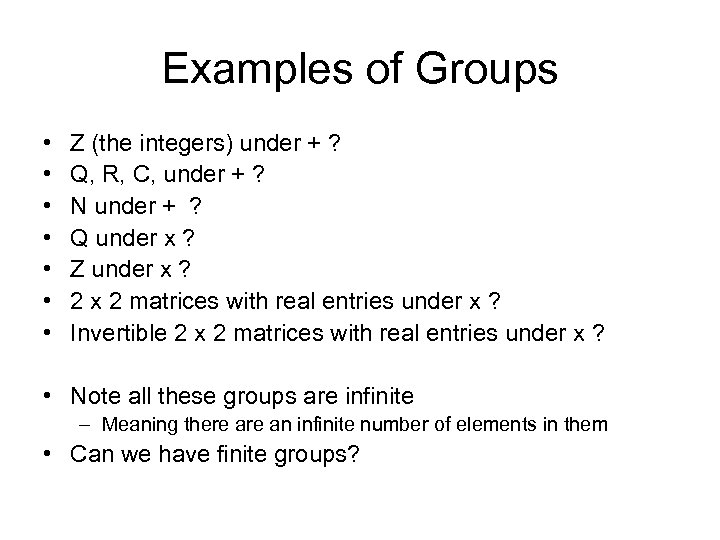Examples of Groups • • Z (the integers) under + ? Q, R, C, under + ? N under + ? Q under x ? Z under x ? 2 x 2 matrices with real entries under x ? Invertible 2 x 2 matrices with real entries under x ? • Note all these groups are infinite – Meaning there an infinite number of elements in them • Can we have finite groups?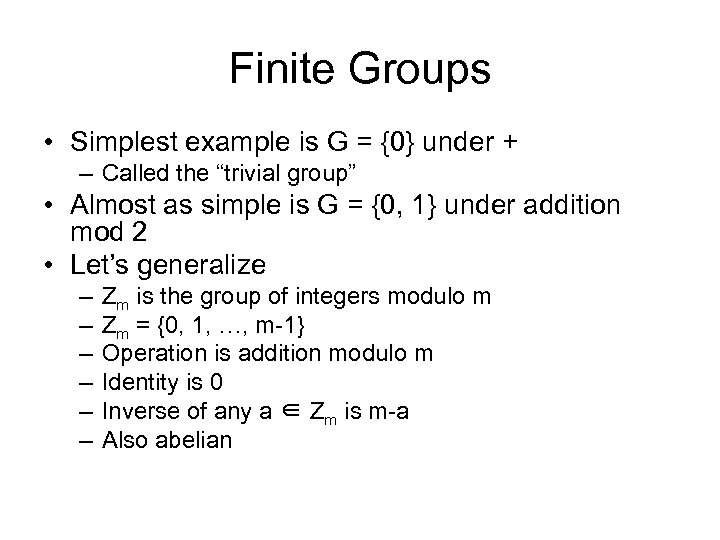Finite Groups • Simplest example is G = {0} under + – Called the “trivial group” • Almost as simple is G = {0, 1} under addition mod 2 • Let’s generalize – – – Zm is the group of integers modulo m Zm = {0, 1, …, m-1} Operation is addition modulo m Identity is 0 Inverse of any a ∈ Zm is m-a Also abelian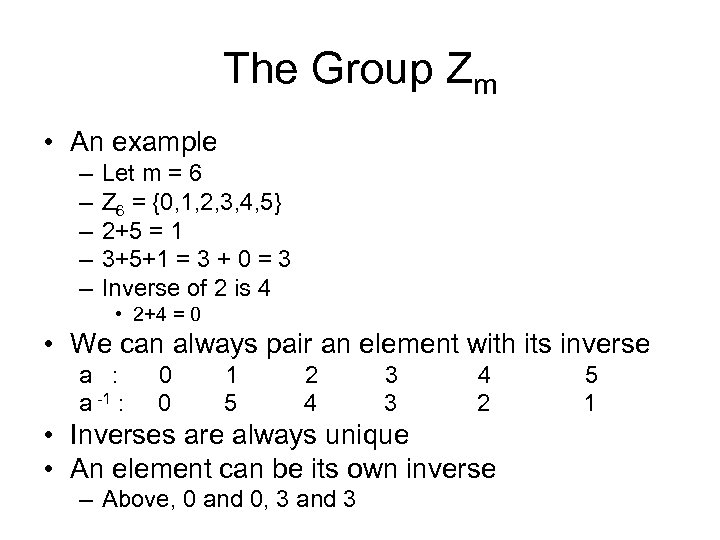The Group Zm • An example – – – Let m = 6 Z 6 = {0, 1, 2, 3, 4, 5} 2+5 = 1 3+5+1 = 3 + 0 = 3 Inverse of 2 is 4 • 2+4 = 0 • We can always pair an element with its inverse a : a -1 : 0 0 1 5 2 4 3 3 4 2 • Inverses are always unique • An element can be its own inverse – Above, 0 and 0, 3 and 3 5 1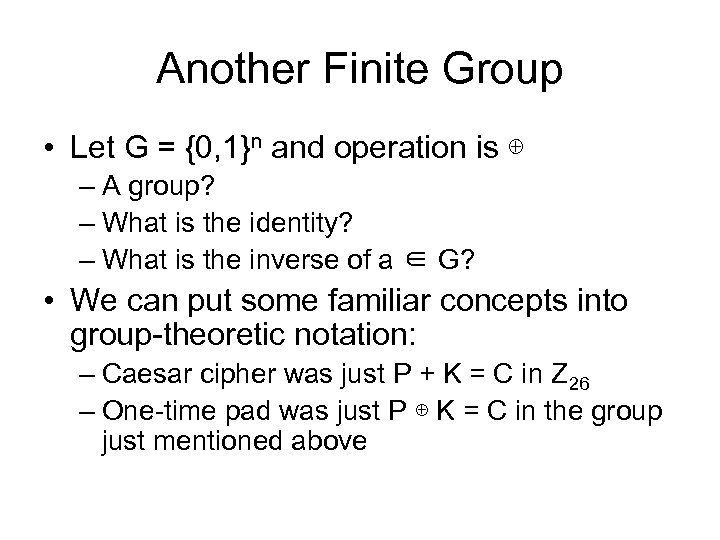Another Finite Group • Let G = {0, 1}n and operation is ⊕ – A group? – What is the identity? – What is the inverse of a ∈ G? • We can put some familiar concepts into group-theoretic notation: – Caesar cipher was just P + K = C in Z 26 – One-time pad was just P ⊕ K = C in the group just mentioned above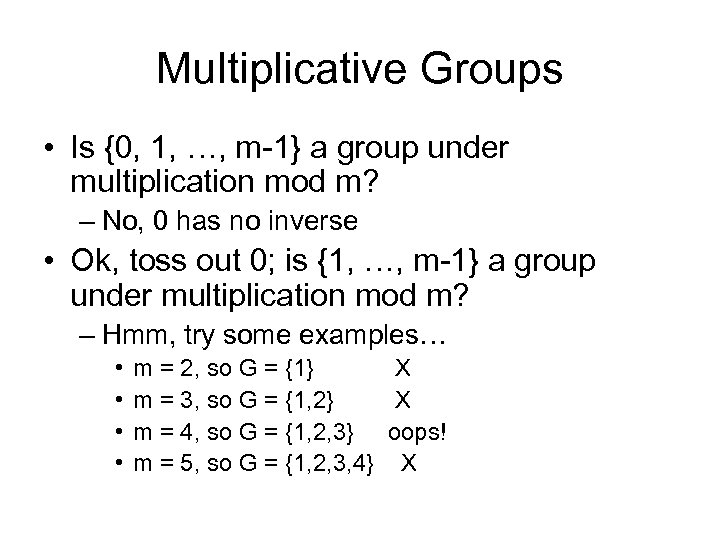Multiplicative Groups • Is {0, 1, …, m-1} a group under multiplication mod m? – No, 0 has no inverse • Ok, toss out 0; is {1, …, m-1} a group under multiplication mod m? – Hmm, try some examples… • • m = 2, so G = {1} X m = 3, so G = {1, 2} X m = 4, so G = {1, 2, 3} oops! m = 5, so G = {1, 2, 3, 4} X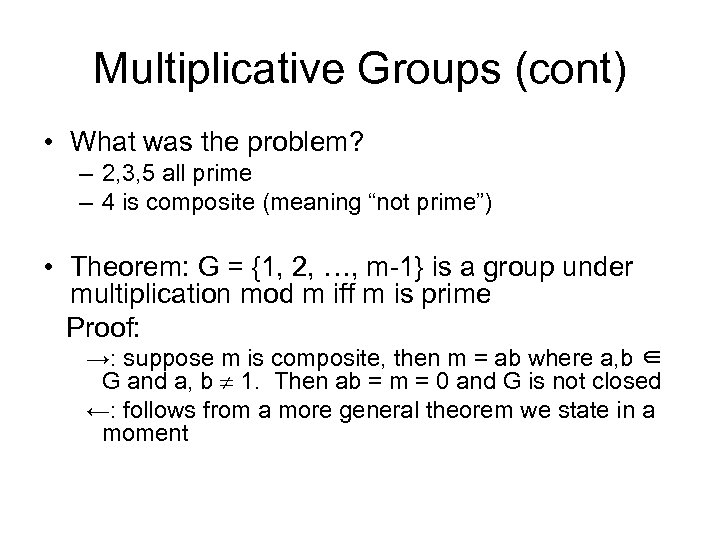Multiplicative Groups (cont) • What was the problem? – 2, 3, 5 all prime – 4 is composite (meaning “not prime”) • Theorem: G = {1, 2, …, m-1} is a group under multiplication mod m iff m is prime Proof: →: suppose m is composite, then m = ab where a, b ∈ G and a, b 1. Then ab = m = 0 and G is not closed ←: follows from a more general theorem we state in a moment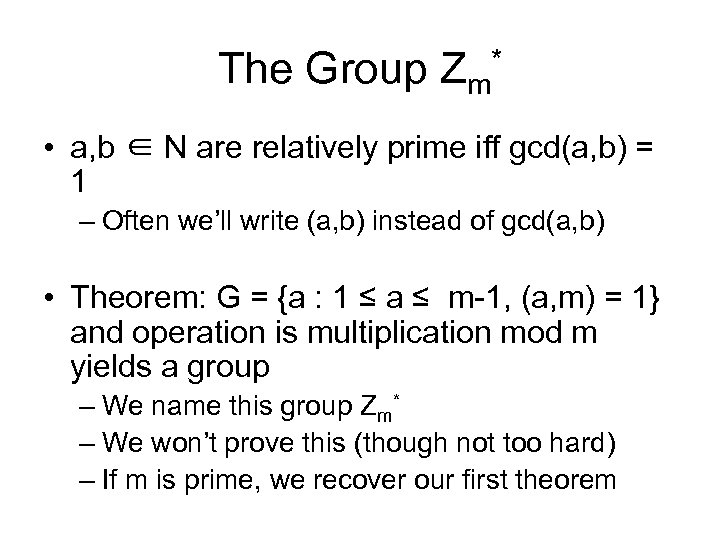The Group Zm* • a, b ∈ N are relatively prime iff gcd(a, b) = 1 – Often we’ll write (a, b) instead of gcd(a, b) • Theorem: G = {a : 1 ≤ a ≤ m-1, (a, m) = 1} and operation is multiplication mod m yields a group – We name this group Zm* – We won’t prove this (though not too hard) – If m is prime, we recover our first theorem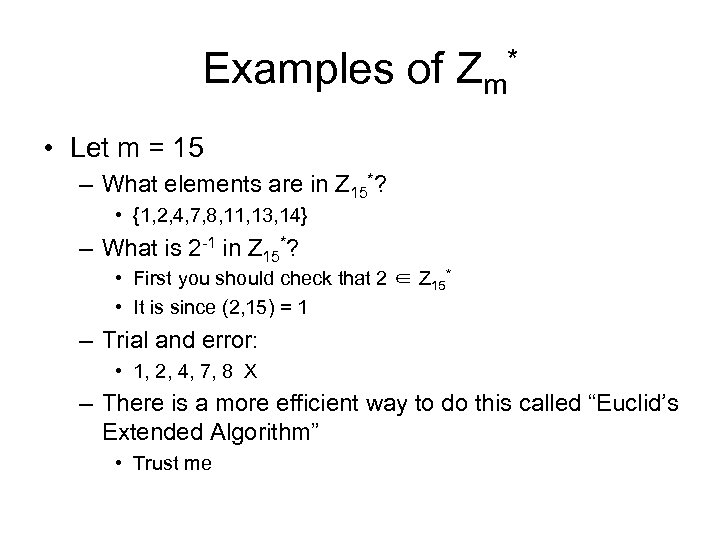Examples of Zm* • Let m = 15 – What elements are in Z 15*? • {1, 2, 4, 7, 8, 11, 13, 14} – What is 2 -1 in Z 15*? • First you should check that 2 ∈ Z 15* • It is since (2, 15) = 1 – Trial and error: • 1, 2, 4, 7, 8 X – There is a more efficient way to do this called “Euclid’s Extended Algorithm” • Trust meEuler’s Phi Function • Definition: The number of elements of a group G is called the order of G and is written |G| – For infinite groups we say |G| = 1 – All groups we deal with in cryptography are finite • Definition: The number of integers i < m such that (i, m) = 1 is denoted (m) and is called the “Euler Phi Function” – Note that |Zm*| = (m) – This follows immediately from the definition of ()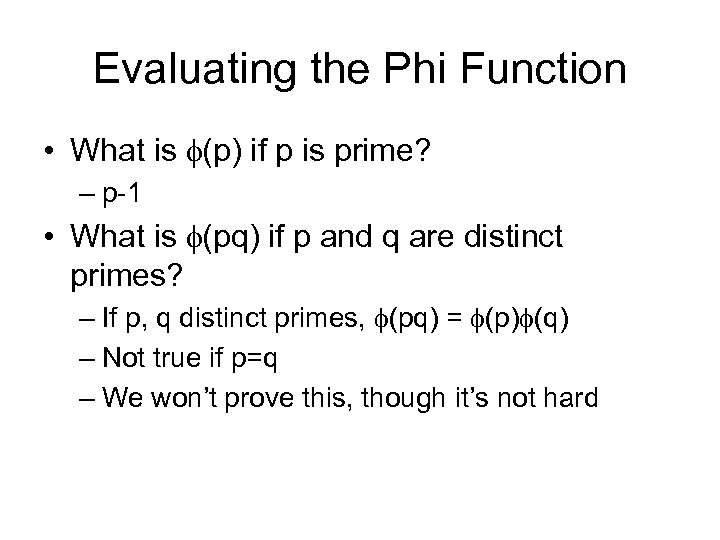Evaluating the Phi Function • What is (p) if p is prime? – p-1 • What is (pq) if p and q are distinct primes? – If p, q distinct primes, (pq) = (p) (q) – Not true if p=q – We won’t prove this, though it’s not hard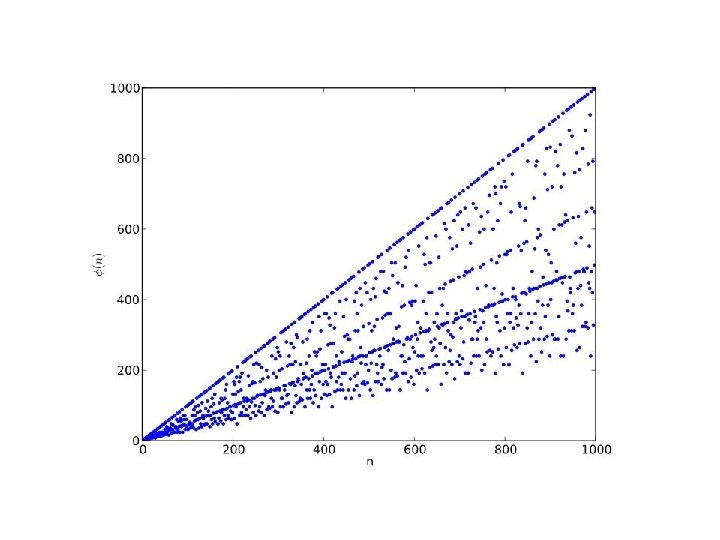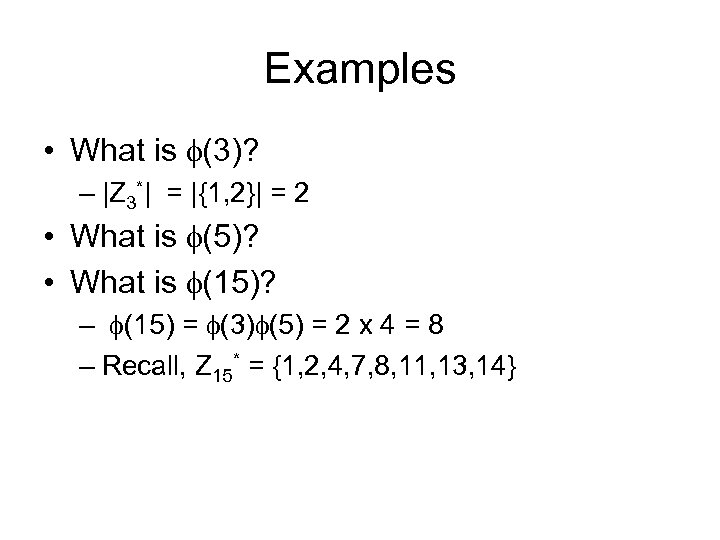Examples • What is (3)? – |Z 3*| = |{1, 2}| = 2 • What is (5)? • What is (15)? – (15) = (3) (5) = 2 x 4 = 8 – Recall, Z 15* = {1, 2, 4, 7, 8, 11, 13, 14}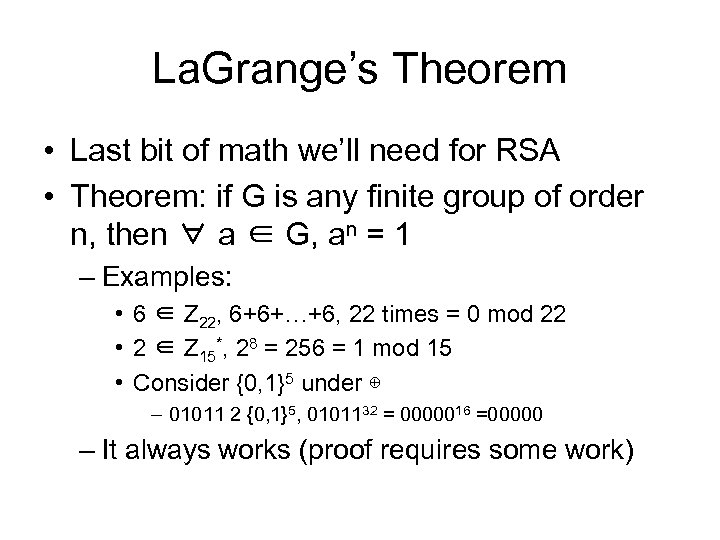La. Grange’s Theorem • Last bit of math we’ll need for RSA • Theorem: if G is any finite group of order n, then ∀ a ∈ G, an = 1 – Examples: • 6 ∈ Z 22, 6+6+…+6, 22 times = 0 mod 22 • 2 ∈ Z 15*, 28 = 256 = 1 mod 15 • Consider {0, 1}5 under ⊕ – 01011 2 {0, 1}5, 0101132 = 0000016 =00000 – It always works (proof requires some work)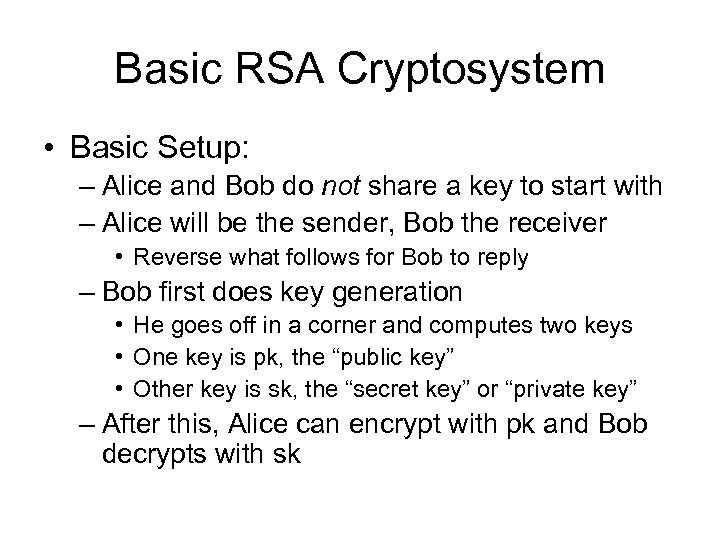Basic RSA Cryptosystem • Basic Setup: – Alice and Bob do not share a key to start with – Alice will be the sender, Bob the receiver • Reverse what follows for Bob to reply – Bob first does key generation • He goes off in a corner and computes two keys • One key is pk, the “public key” • Other key is sk, the “secret key” or “private key” – After this, Alice can encrypt with pk and Bob decrypts with sk